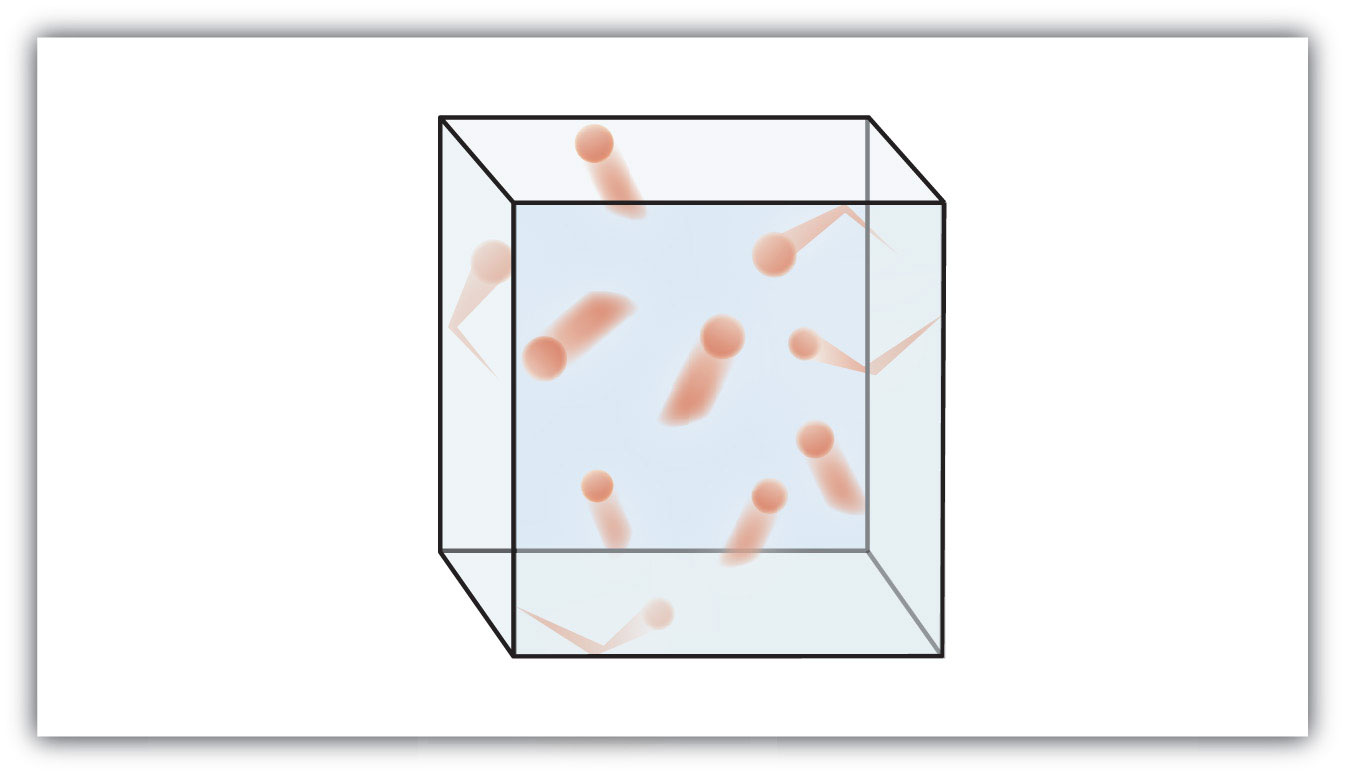# Question #6e2e1

In physical terms, these spheres represent repulsion forces between the different gas particles in a given sample. The spheres themselves are much larger than the gas molecules, and interestingly, the sphere size doesn't change much as the size of the gas molecule changes (assuming ideal behavior). In other words, the volume of the repulsion sphere for a ${H}_{2}$ molecule is virtually identical to that of a ${\text{CH}}_{4}$ molecule. This is why we can approximate the volume occupied by one mole of any gas to be the same (at a constant temperature and pressure) even though the volume of the gas molecules themselves may change.
To clarify, suppose we have a situation as in the image below of red gas molecules contained in a box of volume $V$. It is easy to see that the sum of all the red boxes doesn't come close to the total volume of the box. When we talk about the volume of a gas, we are typically talking about the volume that the gas occupies, in this case the box, $V$.